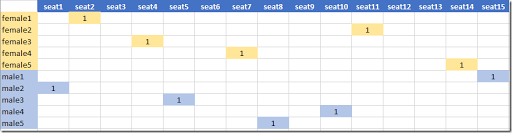## Tuesday, September 20, 2016

### Gender-based Seating Arrangement

From this post:

I'm trying to solve a special assignment problem, but I cannot handle it.

The problem is to assign seats to audiences. Each audience has a ranking of the seats based on his/her own preference. I want to assign the seats to the audiences such that the overall satisfaction is maximized.

The above problem is nothing but an assignment problem, which can be solved by the Hungarian algorithm. Now, things becomes a little more complicated. The audiences are divided into male audiences and female audiences. When assigning the seats, there should be at least n empty seats between each male and female. I assume that there are enough seats to accommodate all audiences and that all seats are located in a row.

This looks like an assignment problem with some side-constraints. The assignment part is boilerplate:

 \boxed{\begin{align}\max\>&\sum_{i,j}s_{i,j}x_{i,j}\\&\sum_j x_{i,j}=1 \>\forall i\\&\sum_i x_{i,j}\le 1\>\forall j\\&x_{i,j}\in \{0,1\}\end{align}}

Here $$i$$ indicates spectators and $$j$$ refers to seats. Modeling the constraint that females cannot sit next to males is more challenging and interesting (from a strict modeling perspective that is: these seating arrangements do not look very attractive to me).

To experiment with some formulations I generate some random data for the preferences $$s_{i,j}$$:

If we want to have $$n$$ empty seats in between the males and females, then for $$m=m_F+m_M$$ persons we need at least $$m+n$$ seats.

Probably the easiest is to use a quadratic model. First we introduce a distance matrix $$d_{j,j’}$$ indicating 1 + the number of seats between seats $$j$$ and $$j’$$. I.e. this matrix looks like: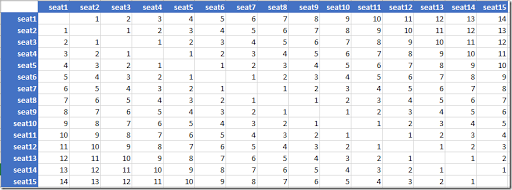Then we need to construct a set $$check_{i,i’}$$ which has all unique female-male combinations we need to check. This set arranged as a matrix looks like: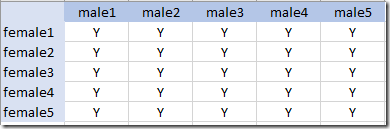With this data we can now write our side-constraint as:

 $\sum_{j\ne j’} d_{j,j’} x_{i,j} x_{i’,j’} \ge n+1 \>\> \forall check(i,i’)$

i.e.: for every combination $$(i,i’)$$ where $$i$$ is a female and $$i’$$ is a male, placed in seats $$(j,j’)$$, make sure the distance $$d_{j,j’}$$ of their seats is at least $$n+1$$.

This formulation is quadratic but unfortunately not convex (the Q matrix of quadratic coefficients is not negative semidefinite). We are lucky: some solvers like Cplex and Gurobi accept it nevertheless: they apply some reformulations behind the scenes. Gurobi really, really wants to let you know and prints:

 Modified 30 Q diagonalsModified 30 Q diagonalsModified 30 Q diagonalsModified 30 Q diagonalsModified 30 Q diagonalsModified 30 Q diagonalsModified 30 Q diagonalsModified 30 Q diagonalsModified 30 Q diagonalsModified 30 Q diagonalsModified 30 Q diagonalsModified 30 Q diagonalsModified 30 Q diagonalsModified 30 Q diagonalsModified 30 Q diagonalsModified 30 Q diagonalsModified 30 Q diagonalsModified 30 Q diagonalsModified 30 Q diagonalsModified 30 Q diagonalsModified 30 Q diagonalsModified 30 Q diagonalsModified 30 Q diagonalsModified 30 Q diagonalsModified 30 Q diagonals

The optimal assignment for $$n=1$$ looks like: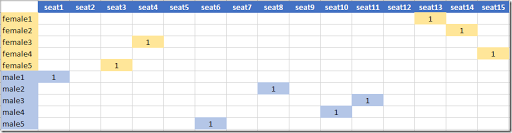##### Linearization

The multiplication of two binary variables can be easily linearized. A standard way to linearize $$z=x\cdot y$$ with $$x,y \in \{0,1\}$$ is:

 \boxed{\begin{align}&z \le x\\ &z \le y \\ & z\ge x+y-1\\& x,y,z \in \{0,1\}\end{align}}

In our case we can simplify a bit:

 \boxed{\begin{align}&\sum_{j,j’} d_{j,j’} y_{i,j,i’,j’} \ge n+1\\ & y_{i,j,i’,j’} \le x_{i,j}\\ & y_{i,j,i’,j’} \le x_{i’,j’}\\ & y_{i,j,i’,j’} \in \{0,1\} \end{align}}

This formulation leads to a very large model: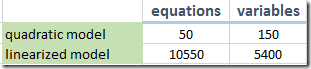Although this linearized formulation finds the optimal solution, clearly, this is not the way to go.

##### Forbid patterns

A different formulation can be based on forbidding certain patterns in the seating. For $$n=1$$ we do not allow F,M or M,F to appear.

The first thing we do is to introduce a variable that tells us if set $$j$$ is occupied by a male or female (or empty). We can do this by using two binary variables $$m_j$$ and $$f_j$$, or we can use a single variable $$g_{j,fm} \in \{0,1\}$$ where $$fm = \{f,m\}$$ is a set indicating the gender.

To calculate $$g_{j,fm}$$ we can use a simple summation over the females or males only, depending on what $$fm$$ is:

 $g_{j,fm}=\sum_{i|gender_{i,fm}} x_{i,j}$

Variable $$g_{i,fm}$$ will look like: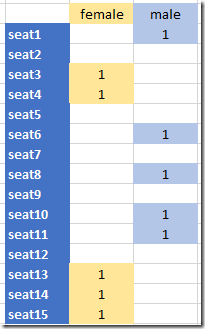This is kind of an aggregation of the variable $$x_{i,j}$$. Based on $$g_{j,fm}$$ we can formulate a set of equations, again assuming $$n=1$$:

 \boxed{\begin{align}&g_{seat1,female}+g_{seat2,male}\le1\\&g_{seat1,male}+g_{seat2,female}\le1\\&g_{seat2,female}+g_{seat3,male}\le1\\&g_{seat2,male}+g_{seat3,female}\le1\\&g_{seat3,female}+g_{seat4,male}\le1\\&g_{seat3,male}+g_{seat4,female}\le1\\&\dots\end{align}}

Of course I did not write these individual equations. Rather I created and populated the 4 dimensional set $$pattern_{k,j,j’,fm}$$ and introduced just one equation block:

 $\sum_{j’,fm|pattern_{k,j,j’,fm}} g_{j’,fm} \le 1 \>\>\forall j,k$

This linear formulation has 83 variables and 180 equations and solves very fast. For $$n>1$$ we can formulate generalizations of this scheme.

##### Different problem: no males next to each other

A slightly different problem is actually easier to formulate: make sure no males are sitting next to each other (because they start to fight?). Also let’s apply the same rule for the women. Again we can use the scheme where we forbid patterns. Here we forbid F,F and M,M.  This we can do by only allowing up to one F or M in two consecutive seats.

First we create a simple set that gives us the groups of two seats next to each other. Call this set $$group_{j,j’}$$. It looks like: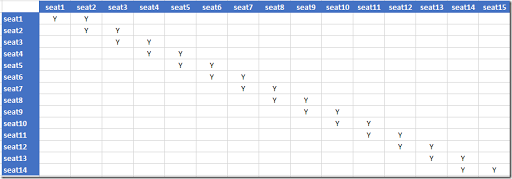We can formulate the spacing equation simply as:

 $\sum_{i|gender_{i,fm}} \sum_{j’|group_{j,j’}} x_{i,j’} \le 1\>\> \forall j,fm$

Actually we can improve on this a little bit. Using extra variables $$g_{j,fm}$$ indicating the gender of the person in seat $$j$$ as defined in the previous paragraph we can write:

 \begin{align}&g_{j,fm}=\sum_{i|gender_{i,fm}} x_{i,j}\>\> \forall j,fm\\&\sum_{j’|group_{j,j’}} g_{j’,fm} \le 1\>\>\forall j,fm \end{align}

Although we add extra variables and equations, this makes the model more readable, and actually sparser (i.e. fewer non-zero elements). Often larger and sparser is better than smaller and denser.

The results looks like: